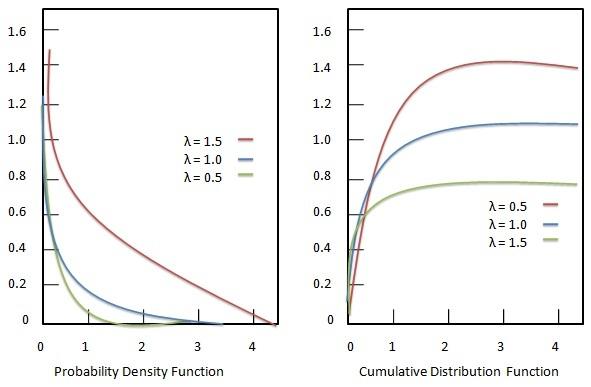# Statistics - Exponential distribution

Exponential distribution or negative exponential distribution represents a probability distribution to describe the time between events in a Poisson process. In Poisson process events occur continuously and independently at a constant average rate. Exponential distribution is a particular case of the gamma distribution.### Probability density function

Probability density function of Exponential distribution is given as:

## Formula

${ f(x; \lambda ) = }$ $\begin {cases} \lambda e^{-\lambda x}, & \text{if$x \ge 0 $} \\[7pt] 0, & \text{if$x \lt 0 $} \end{cases}$

Where −

• ${\lambda}$ = rate parameter.

• ${x}$ = random variable.

### Cumulative distribution function

Cumulative distribution function of Exponential distribution is given as:

## Formula

${ F(x; \lambda) = }$ $\begin {cases} 1- e^{-\lambda x}, & \text{if$x \ge 0 $} \\[7pt] 0, & \text{if$x \lt 0 $} \end{cases}$

Where −

• ${\lambda}$ = rate parameter.

• ${x}$ = random variable.